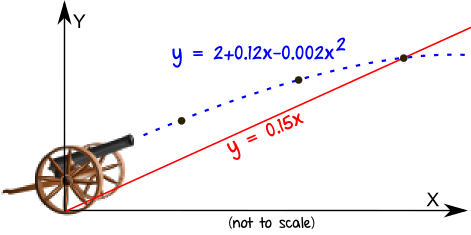By the end of this topic you will be able to:
• Given the nature of the roots of a quadratic equation, use the discriminant to find an unknown
• Finding the coordinates of the point(s) of intersection of a straight line and a curve or of two curves
• know condition for tangency; intersection of line and parabola (lines and curves)